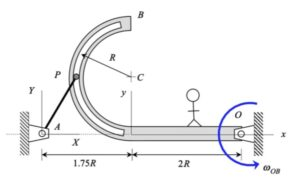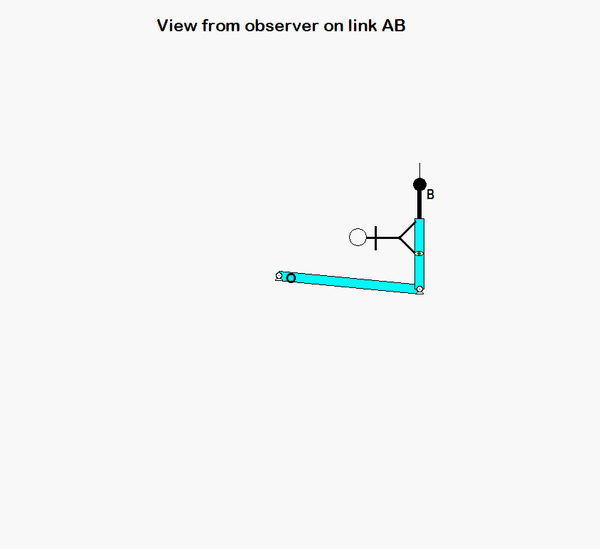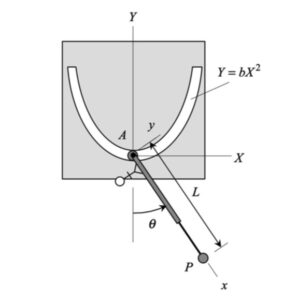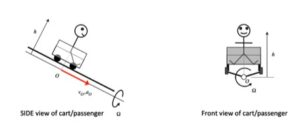# Homework H.4.B# Homework H.3.G# Homework H.3.H# Homework H.3.E# Homework H.3.F# Homework H.3.CDiscussionShown above is the motion of the mechanism as seen by a fixed observer.For the same motion, the above animation shows the view of an observer that is attached to the slotted link.

It is suggested that you employ the view of the observer on the slotted arm in your analysis.

# Homework H.3.DDiscussion• Shown above left is the motion of the mechanism as seen by a fixed observer. Note that the stationary observer sees P moving on a circular path centered at point A.
• And, shown above right is the motion of P as seen by an observer attached to the slotted arm. Note that the moving observer sees P moving on a circular path centered at point C.

It is suggested that you employ the view of the observer on the slotted arm in your analysis.

# Homework H.3.ADiscussionAn animation from the simulation of the motion of the robotic arm system is shown above for a fixed observer. The motion of end-effector B is quite complicated, as can be seen. However, if we attach an observer to section AD of the end link of the arm, the observed motion of B is greatly simplified. In fact, the observer simply sees a back-and-forth motion of B in the x-direction, as shown in the animation below.HINTS: The velocity of point B can be found from the following moving reference frame velocity equation:

vB = vA + (vB/A)rel + ω × rB/A

vA = (L θ_dot) i
ω
= φ_dot k
(vB/A)rel = b_dot i

Use similar logic for writing down the acceleration of B. Be careful in writing down the expression for aA ; in particular, a0. (A is traveling on a circular path with a constant speed.)

# Homework H.3.B